Related Articles

# How to Use Select Case Statement in Excel VBA?

• Last Updated : 19 Jul, 2021

VBA in Excel stands for Visual Basic for Applications which is Microsoft’s programming language. To optimize the performance and reduce the time in Excel we need Macros and VBA is the tool used in the backend.

In this article, we are going to discuss how to use Select Case Statement in Excel VBA.

### Implementation :

In the Microsoft Excel tabs, select the Developer Tab. Initially, the Developer Tab may not be available.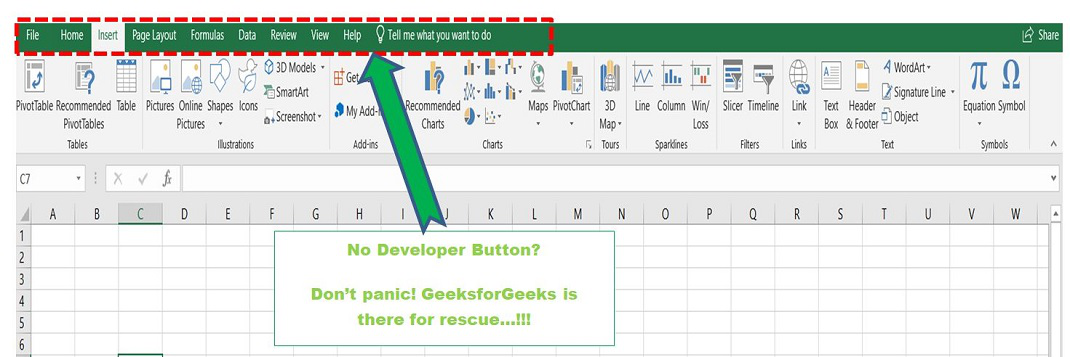The Developer Tab can be enabled easily by a two-step process :

• Right-click on any of the existing tabs at the top of the Excel window.
• Now select Customize the Ribbon from the pop-down menu.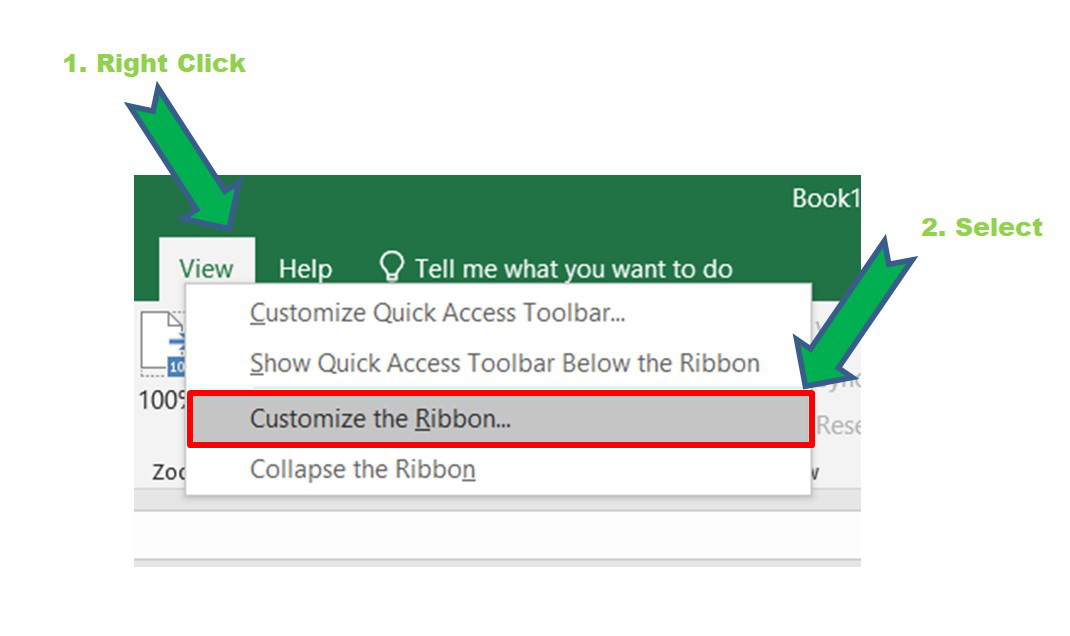• In the Excel Options Box, check the box Developer to enable it and click on OK.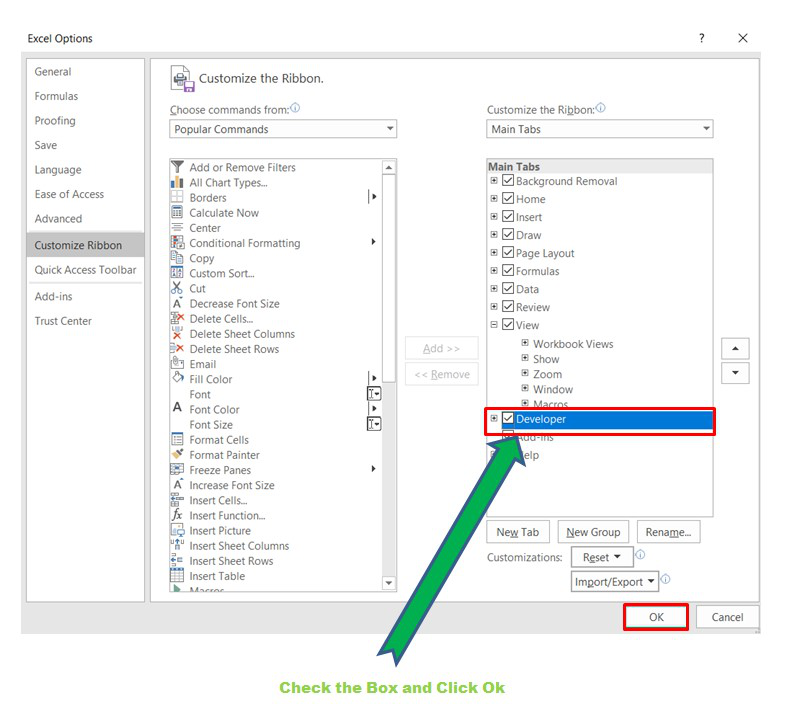• Now, the Developer Tab is visible.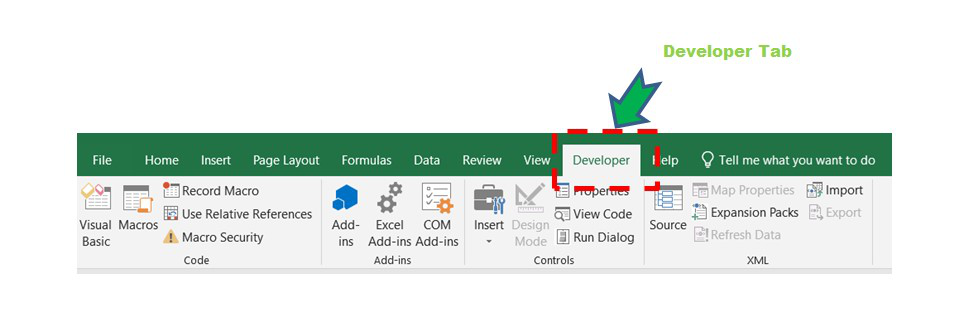• Now click on the Visual Basic option in the Developer tab and make a new module to write the program using the Select Case statement.
`Developer  -> Visual Basic -> Tools -> Macros`
• Now create a Macro and give any suitable name.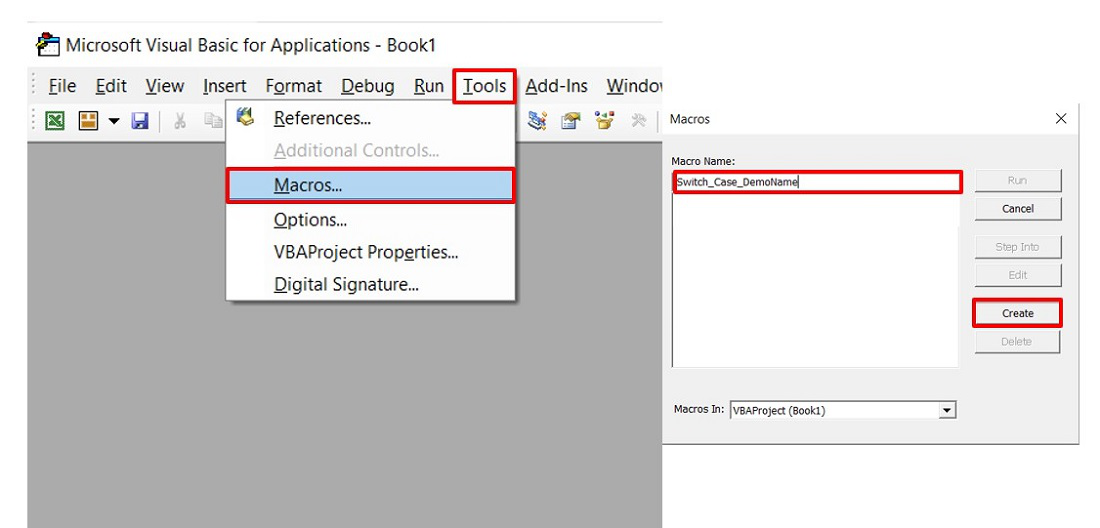• This will open the Editor window where can write the code.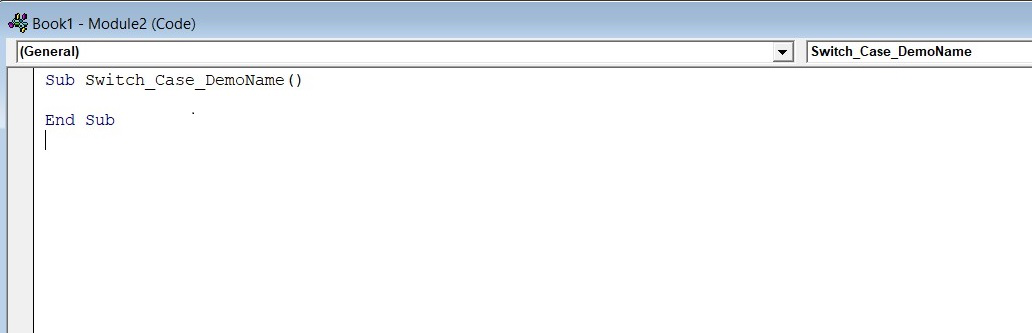## Select Case Statement:

The select case statement is similar to SWITCH-CASE statement in programming languages like C,C++, JAVA, etc. The structure of Select Case in Excel is :

```Select Case Expression/Condition
Case Val_1
Block of statements when Expression matches Val_1
Case Val_2
Block of statements when Expression matches Val_2
Case Val_3
Block of statements when Expression matches Val_3
.
.
.
Case Else
Block of code when none of the above conditions match
End Select

Val_1, Val_2,... are the values.```

Some important keywords used in Select Case in Excel are as follows :

• Case Is: It is basically used with numbers.

For example Case IS < 70 // Means all numbers less than 70.

• Case Else: If none of the values of Cases matches with the Expression. It is similar to the default in the SWITCH statement in C/C++.
•  InputBox: To take input from the user.
•  MsgBox: To display output to the user.

Example 1 :

We want to display the grades of students as per the marks obtained by them in an exam. Consider the data set shown below :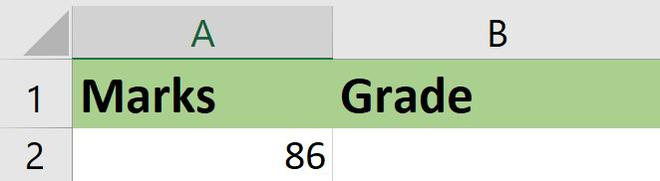Code :

```Sub Select_Case_Grade()
'Declaring variables to fetch marks and store the grade
Dim marks As Integer, Grade As String
'Fetching marks from the Excel cell
marks = Range("A2").Value
Select Case marks
Case Is >= 90
Case Is >= 80
Case Is >= 70
Case Is >= 60
Case Is >= 50
Case Is >= 40
result = "E"
Case Else
End Select
'Displaying the grade in the Excel cell
End Sub```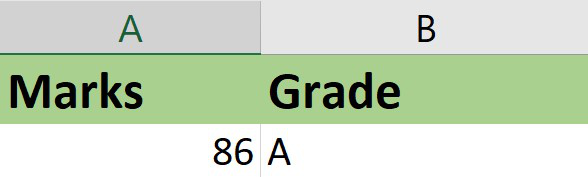Now, change the marks the Grade displayed will be “S”.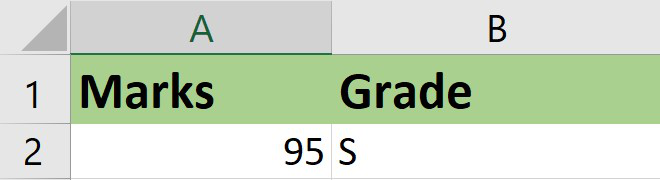You can also write the previous code using range of numbers instead of Case Is

```Sub Select_Case_Grade()
'Declaring variables to fetch marks and store the grade
Dim marks As Integer, Grade As String
'Fetching marks from the Excel cell
marks = Range("A2").Value
Select Case marks
Case 91 To 100
Case 81 To 90
Case 71 To 80
Case 61 To 70
Case 51 To 60
Case 40 To 50
result = "E"
Case Else
End Select
'Displaying the grade in the Excel cell
End Sub```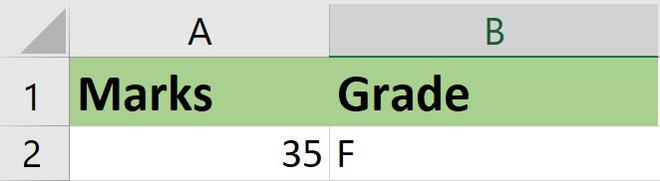Example 2: Consider in a company, employees have to work on a project on the basis of shifts. The company wants to allocate shifts based on odd-even rules and keep age as the deciding criteria. If the age of the employee is odd then he/she has to work in the night shift and if it is even then in the morning shift.

Select Case where the user can input the data in a box.

```Sub Select_Case_Allocate()
'Declaring variables to fetch marks and store the grade
Dim Age As Integer
'Asking the user to enter the age
Select Case (Age Mod 2) = 0
Case True
MsgBox "You will work in the morning shift"
Case False
MsgBox "You will work in the night shift"
End Select
End Sub```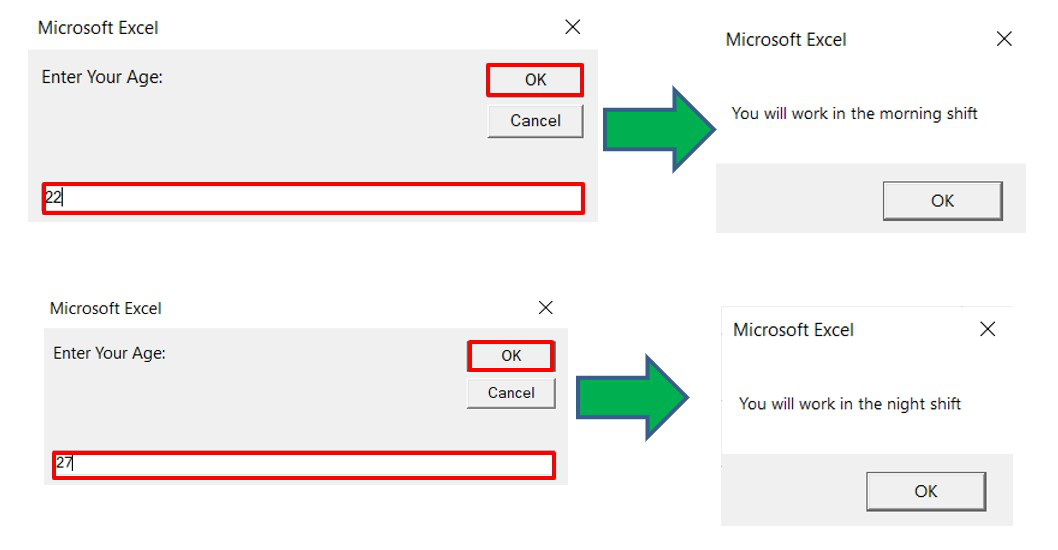Example 3: Let’s create a small calculator which takes two numbers as input and performs addition and multiplication of these numbers.

Code :

```Sub Select_Case_Calculator()
'Declaring variables to fetch marks and store the grade
Dim num1 As Integer, mum2 As Integer, operator As String, res As Integer
'Asking the user to enter the numbers and operator to calculate
num1 = InputBox("Enter The First Number:")
num2 = InputBox("Enter The Second Number:")
operator = InputBox("Enter The Operator Name(Sum,Mul):")
Select Case operator
Case "Sum"
res = num1 + num2
MsgBox ("The result is :" & res)
Case "Mul"
res = num1 * num2
MsgBox ("The result is :" & res)
Case Else
MsgBox "Please Enter a Valid Operator"
End Select
End Sub```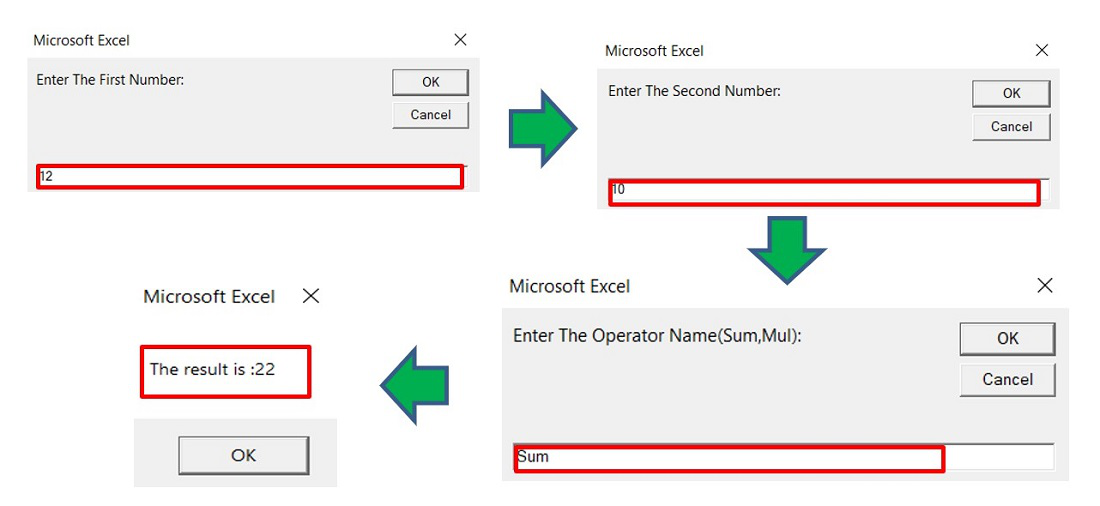We can modify the above code and use multiple conditions in the case. For example, the user can input the string Sum as “SUM” or “sum” as the Excel dialog box is case-sensitive.

```Sub Select_Case_Calculator()
'Declaring variables to fetch marks and store the grade
Dim num1 As Integer, mum2 As Integer, operator As String, res As Integer
'Asking the user to enter the numbers
num1 = InputBox("Enter The First Number:")
num2 = InputBox("Enter The Second Number:")
operator = InputBox("Enter The Operator Name(Sum,Mul):")
Select Case operator
Case "Sum", "SUM", "sum", "SUm", "SuM", "suM", "sUm"
res = num1 + num2
MsgBox ("The result is :" & res)
Case "Mul", "mul", "MUL", "MuL", "muL", "mUl", "MUl"
res = num1 * num2
MsgBox ("The result is :" & res)
Case Else
MsgBox "Please Enter a Valid Operator"
End Select
End Sub```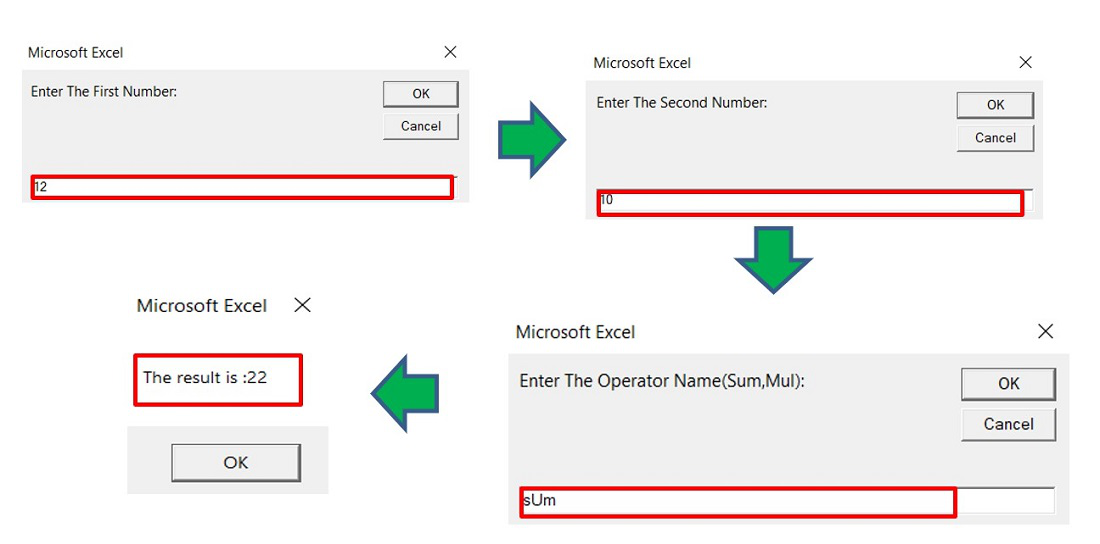Example 4: Let’s see an example using a nested Select Case.

Consider a company that has a policy department-wise regarding the total number of leaves an employee can take in a single year. Now, there are multiple departments and there are female as well as male employees and everyone has different policies for applying for leave. So, a nested Select Case is used to build the problem statement where users can enter the details of department and gender to check the maximum number of days they can take leave in a year.

```Sub Select_Case_Empleave()
'Declaring variables to fetch Department and gender of employee
Dim Department As String, sex As String
'Asking the user to enter the details
sex = InputBox("Enter Your Gender (Male,Female):")
Select Case Department
Case "HR"
Select Case sex
Case "Male"
MsgBox ("You can take maximum 10 days leave in an year")
Case "Female"
MsgBox ("You can take maximum 20 days leave in an year")
Case Else
MsgBox ("Invalid Gender")
End Select
Case "IT"
Select Case sex
Case "Male"
MsgBox ("You can take maximum 15 days leave in an year")
Case "Female"
MsgBox ("You can take maximum 25 days leave in an year")
Case Else
MsgBox ("Invalid Gender")
End Select
Case Else```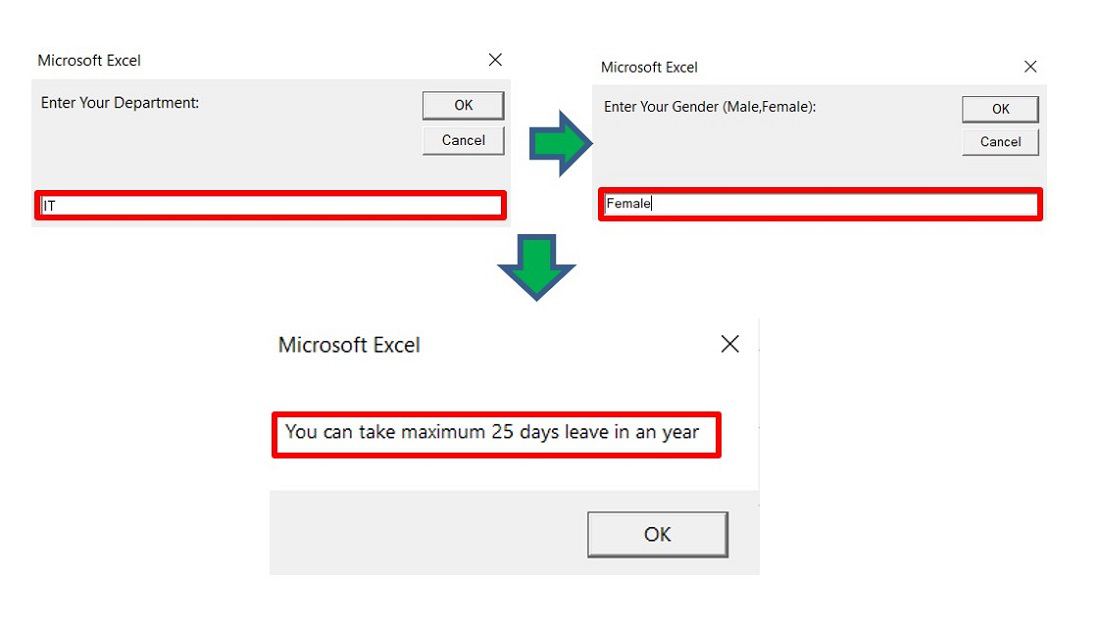Attention reader! Don’t stop learning now. If you are an Excel beginner (or an intermediate) and want to learn Excel, Geeksforgeeks brings the perfect course for you to start, Diving Into Excel

My Personal Notes arrow_drop_up